# RD Sharma Solutions for Class 7 Maths Chapter 21 Mensuration - II (Area of Circle)

Get free PDF of RD Sharma Solutions for Class 7 Maths Chapter 21 Mensuration – II (Area of Circle) from the given links. Students who aim to top the examinations can refer to RD Sharma Solutions. BYJU’S expert team has solved the questions in such a way that learners can understand easily and competently. Learners can simply download the PDF’s from the available links. Chapter 21, Mensuration – II (Area of Circles) includes two exercises. RD Sharma Solutions for Class 7 includes answers to all questions present in these exercises. Let us have a look at some of the concepts that are being discussed in this Chapter.

• Definition of circle
• Circular region
• Chord of a circle
• The diameter of a circle
• Concentric circles
• Congruent circles
• Circumference of a circle
• The relation between diameter and circle
• Area of a circle

## Download the PDF of RD Sharma Solutions For Class 7 Maths Chapter 21 Mensuration – II (Area of Circle)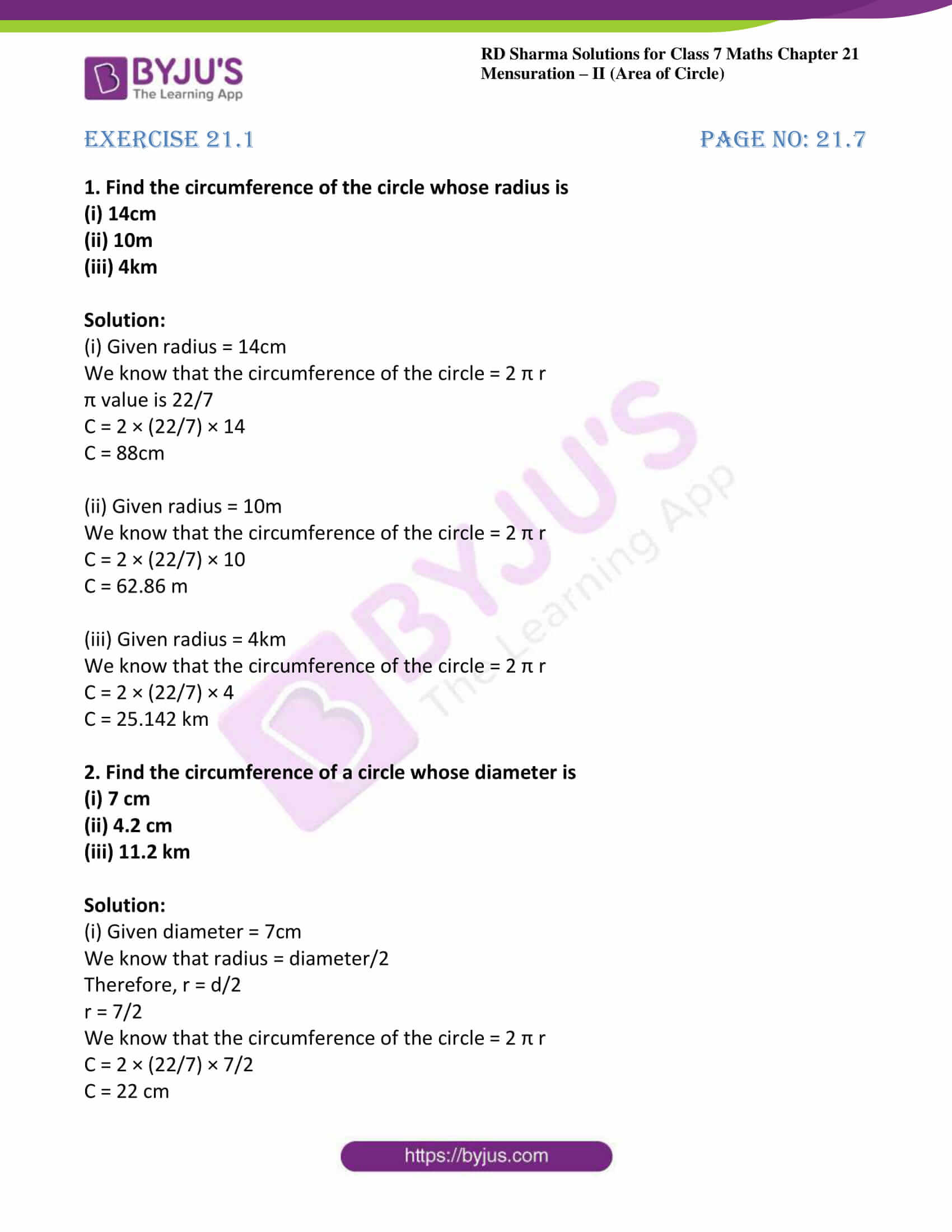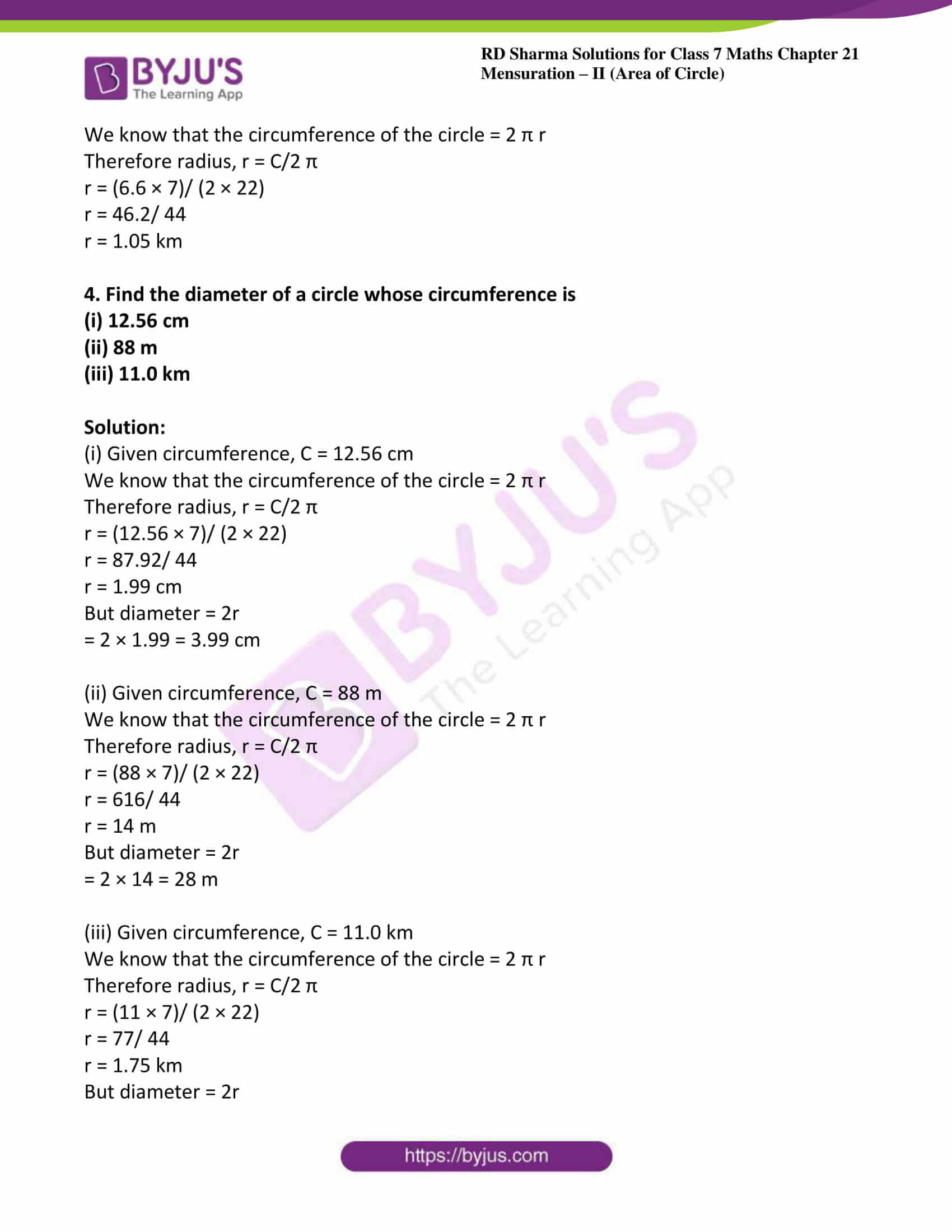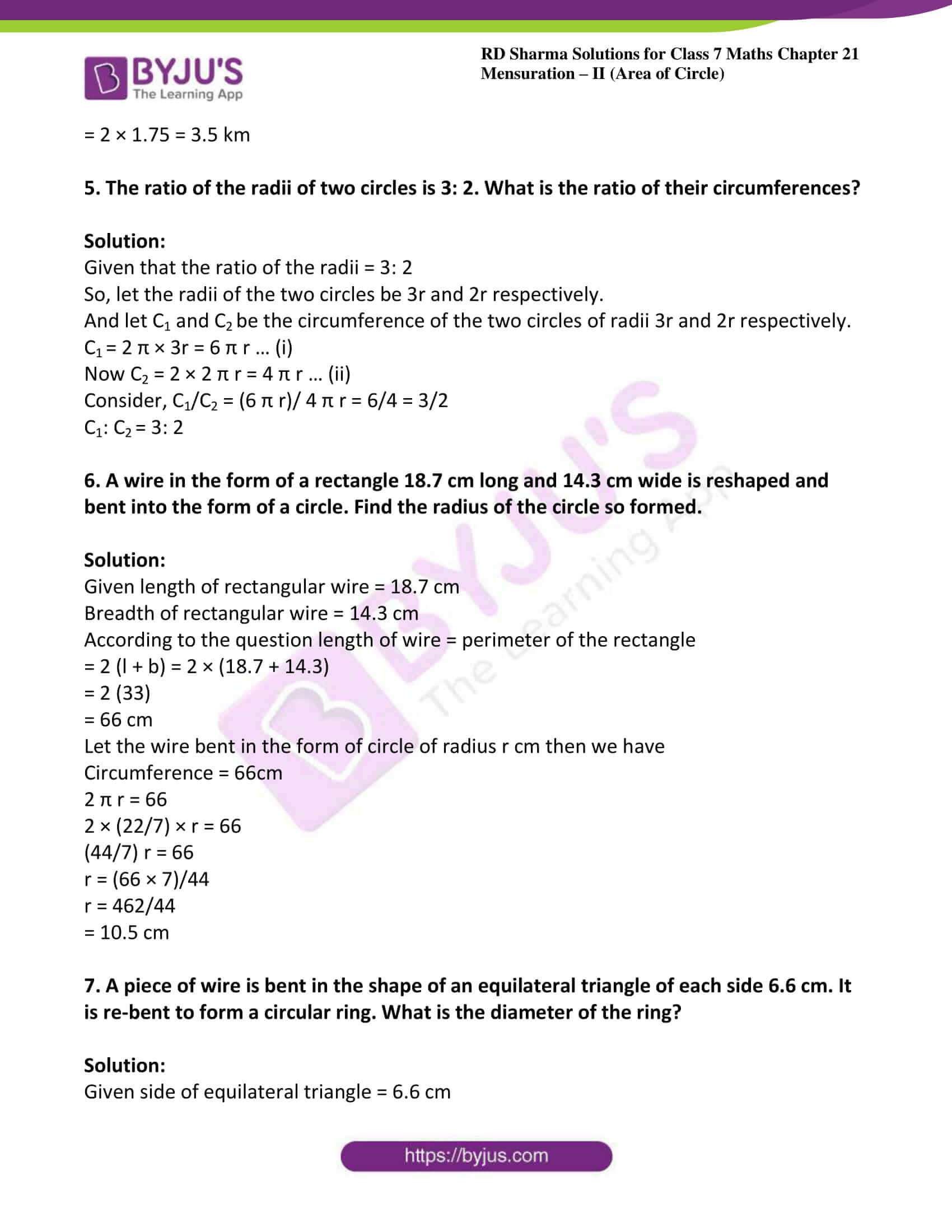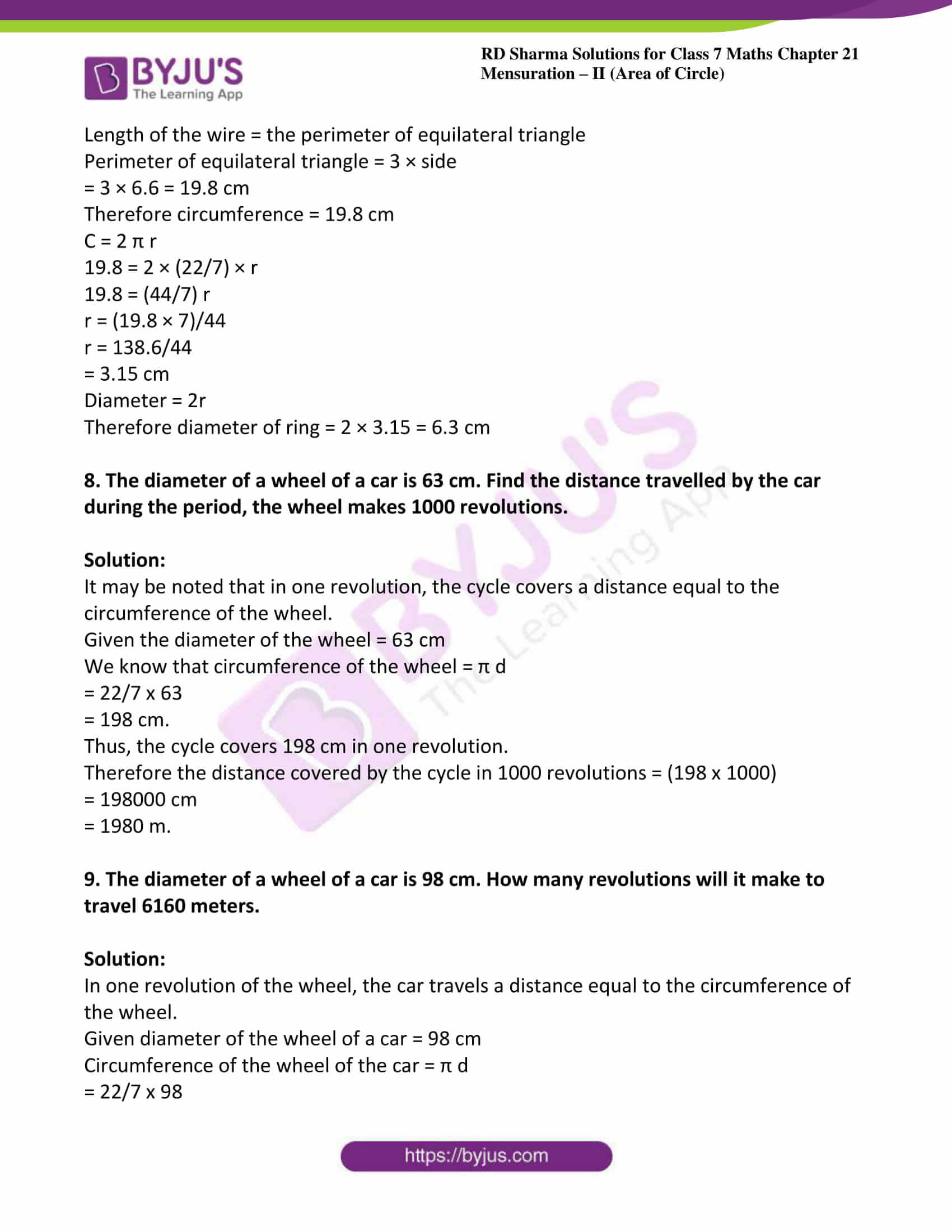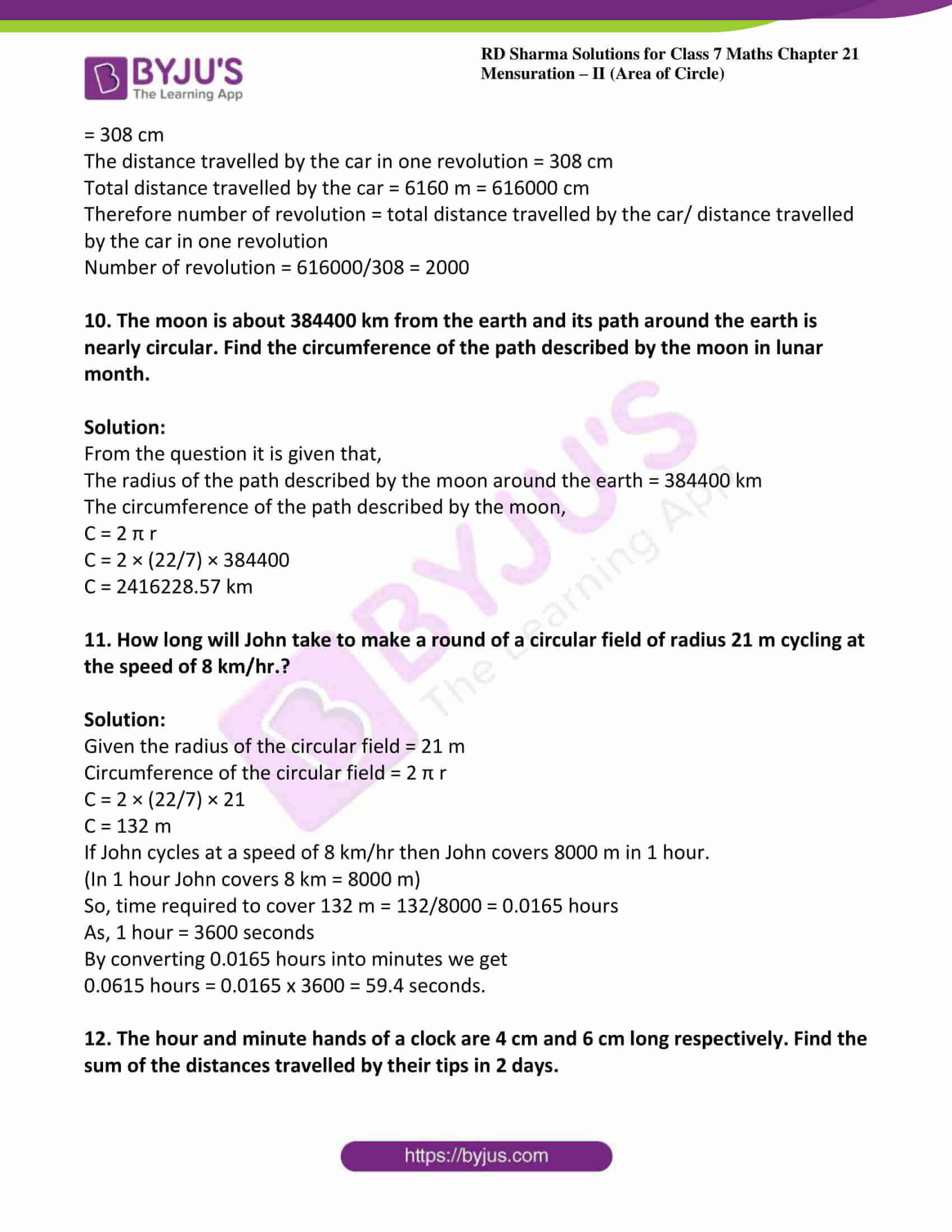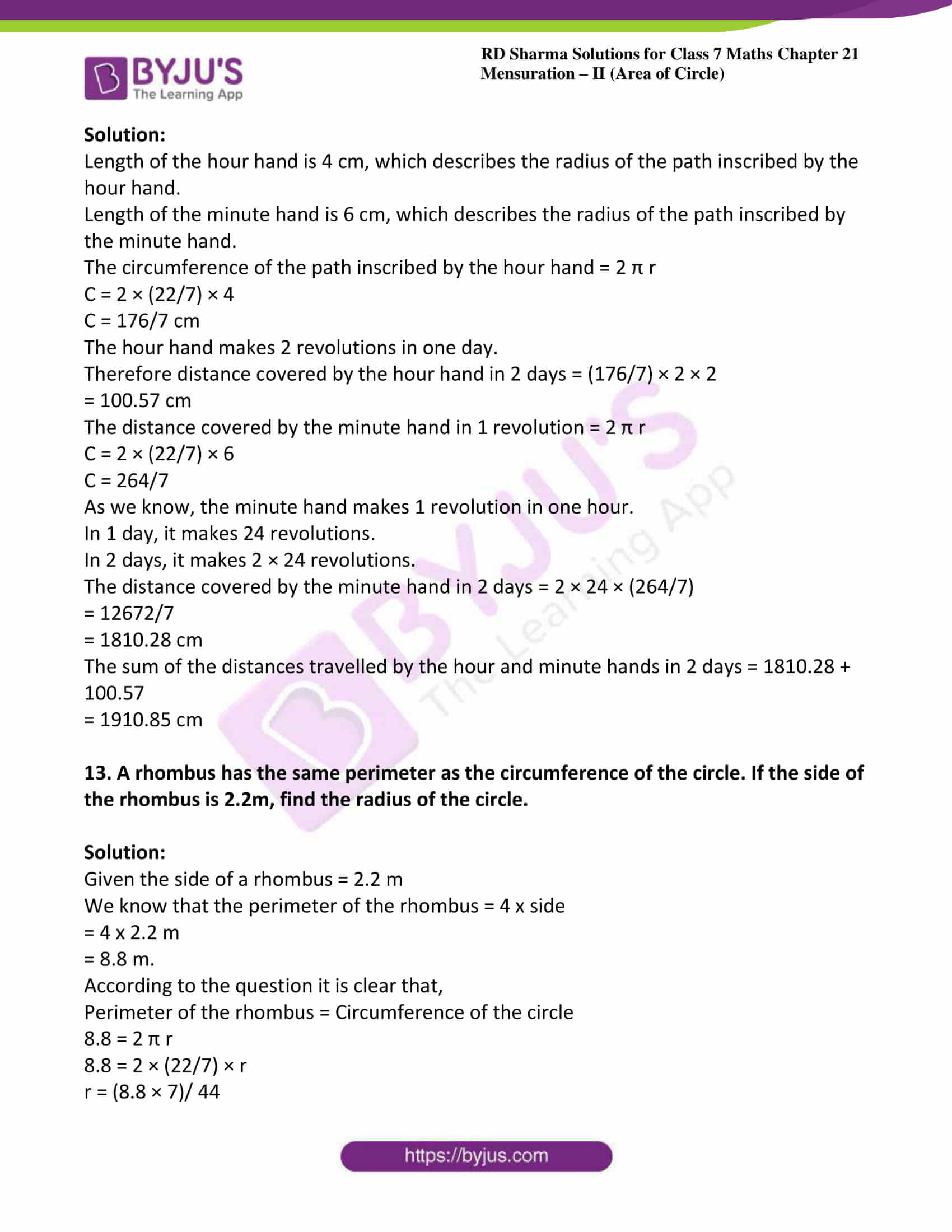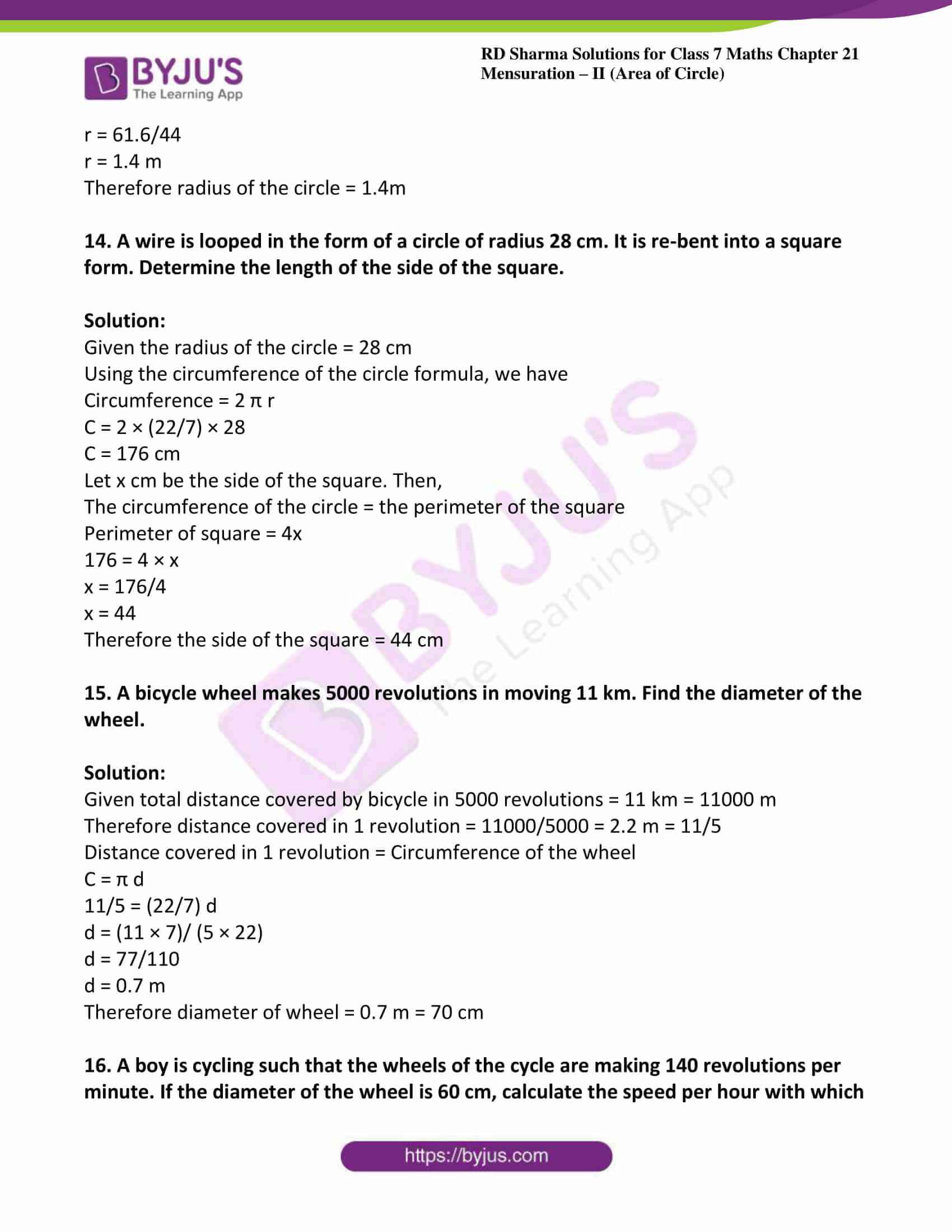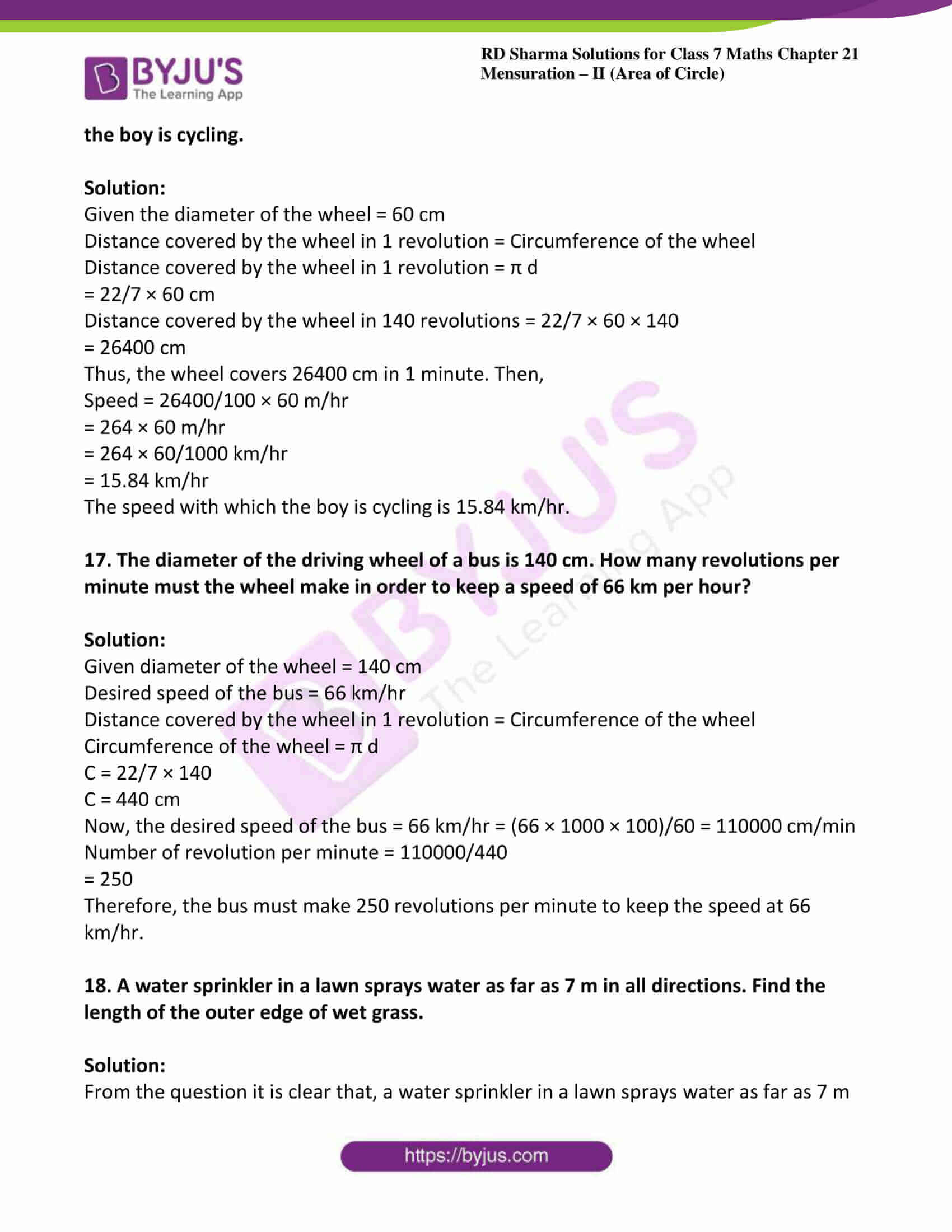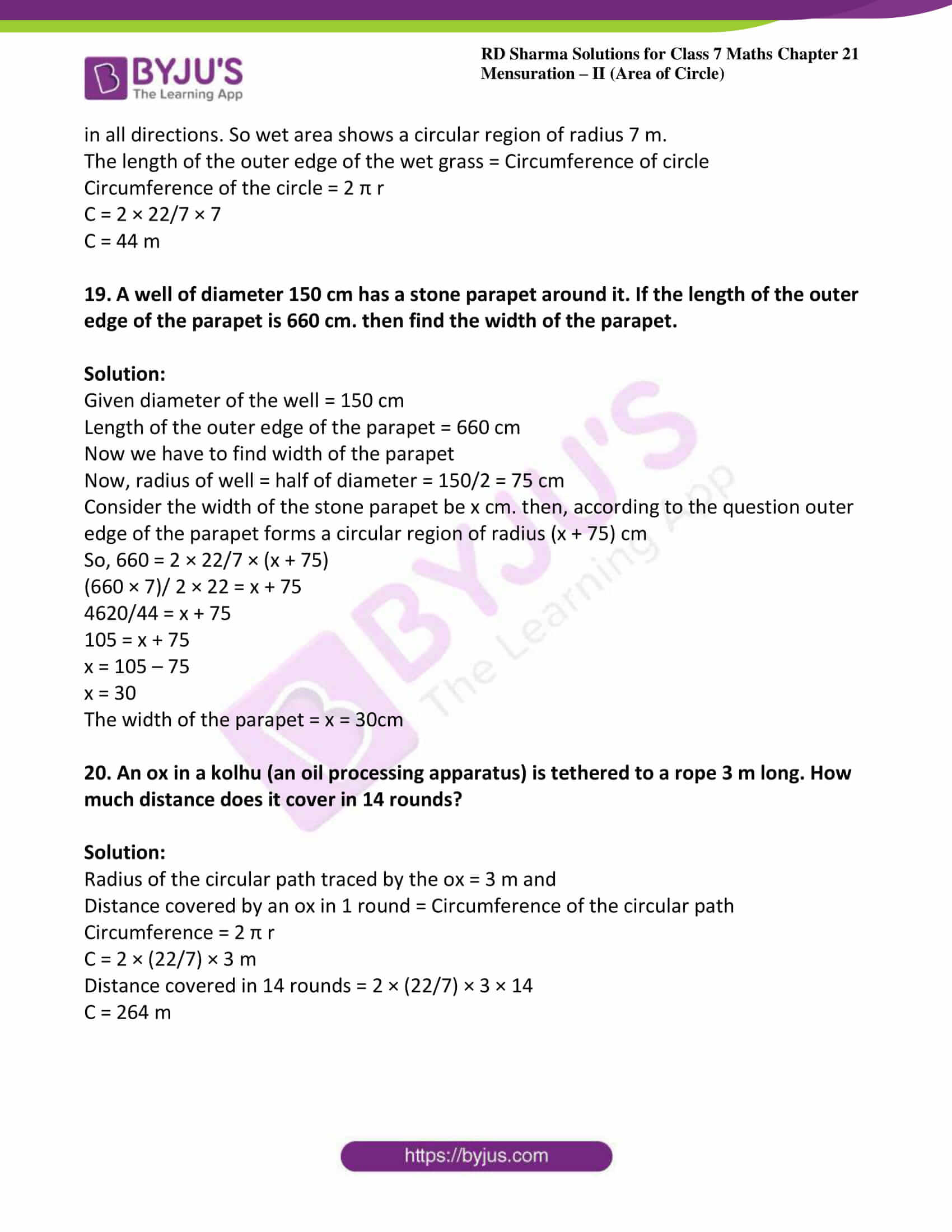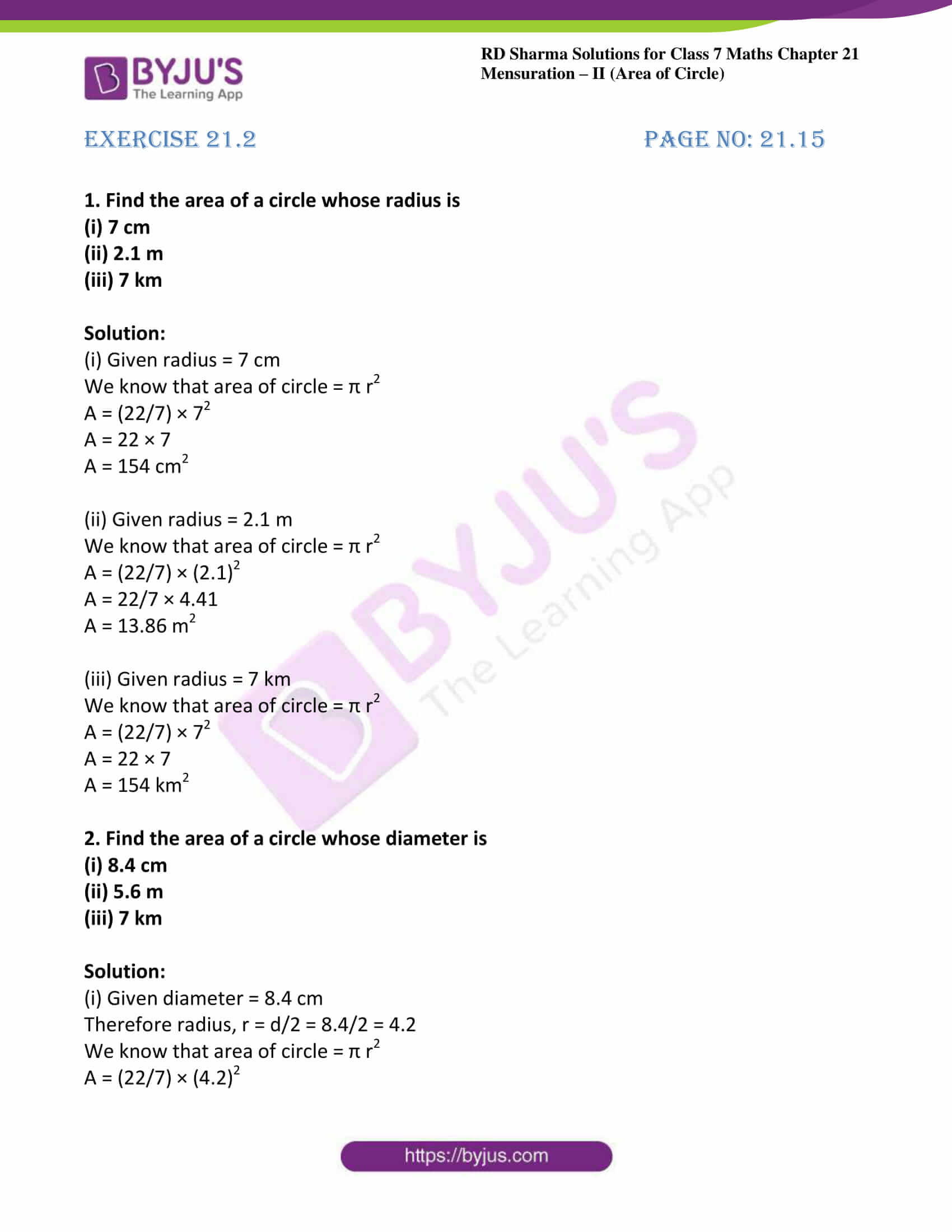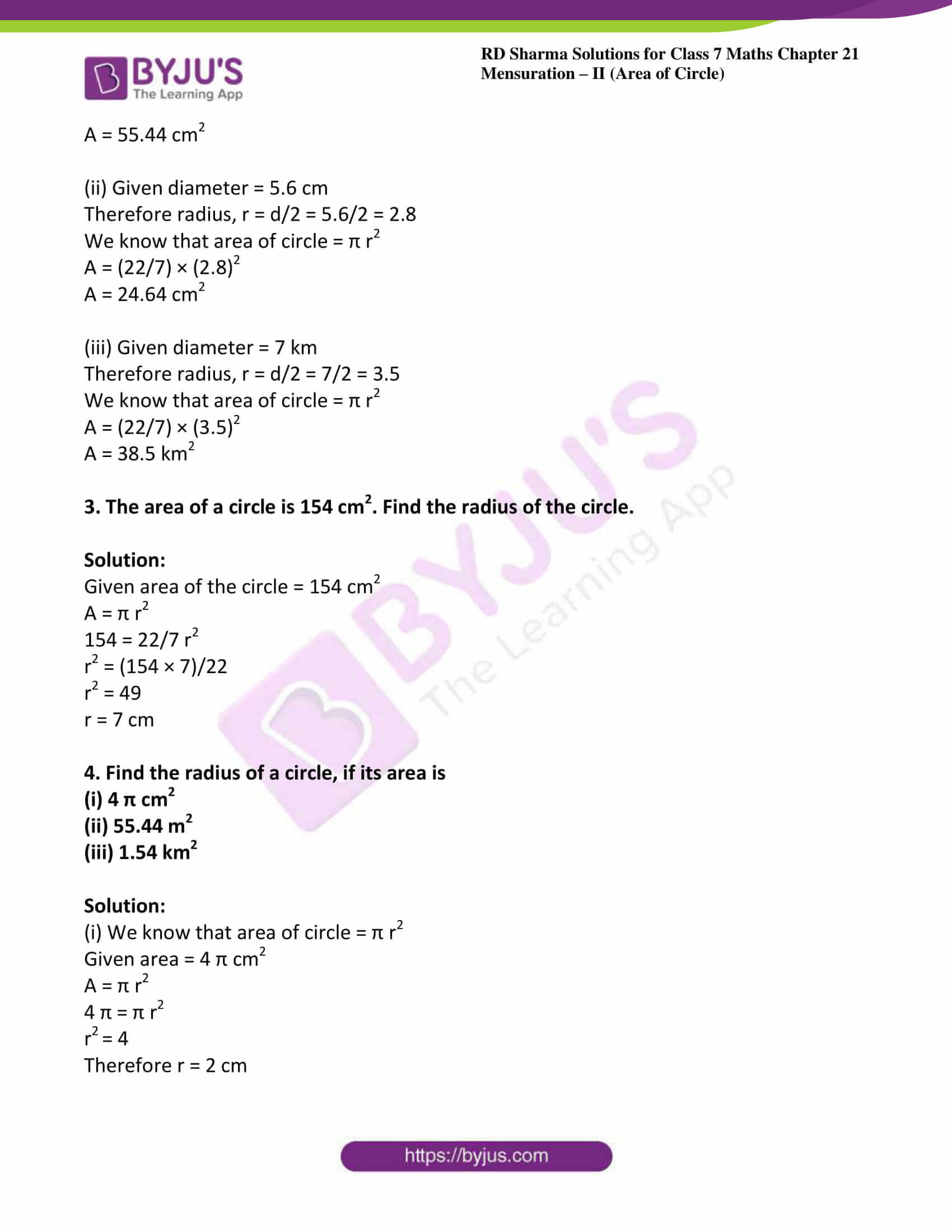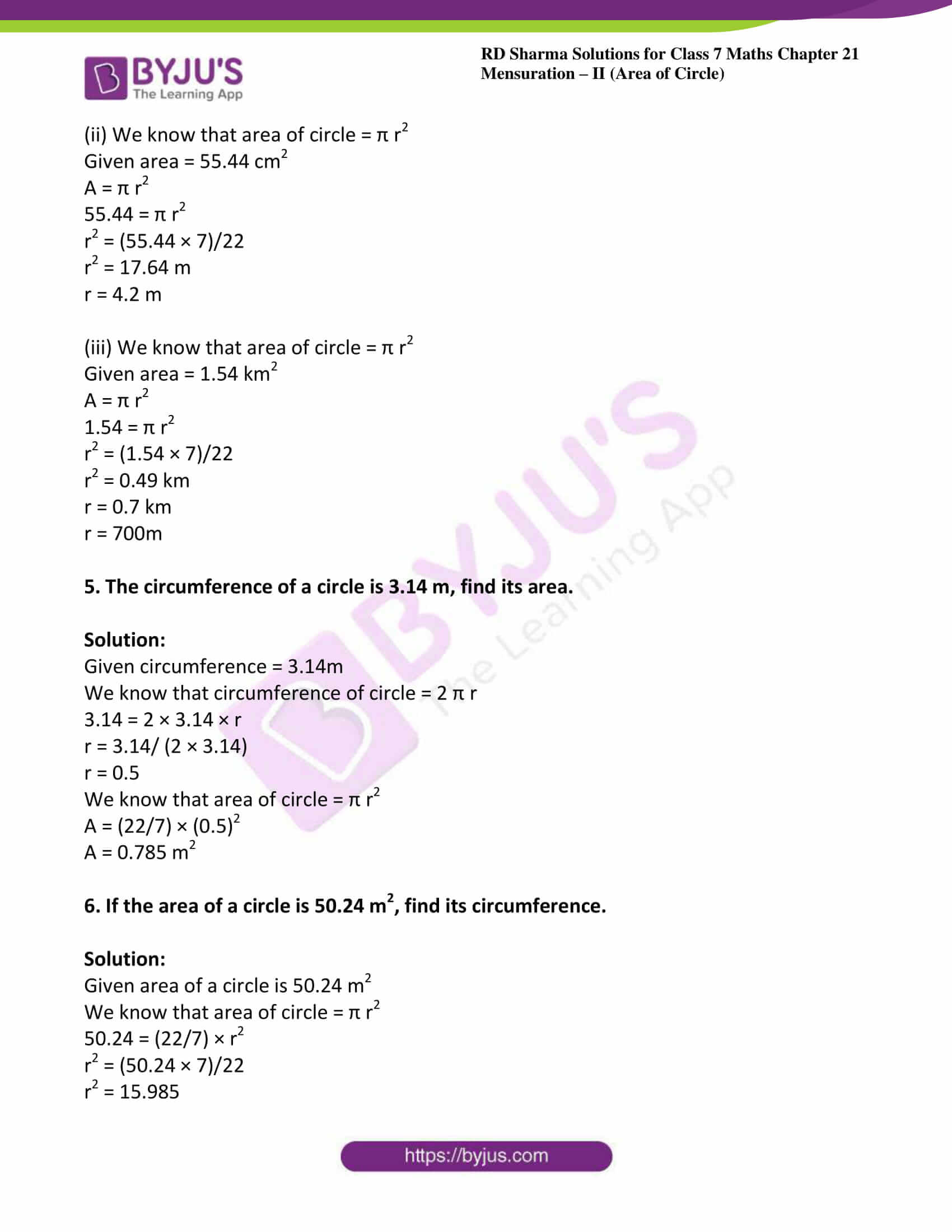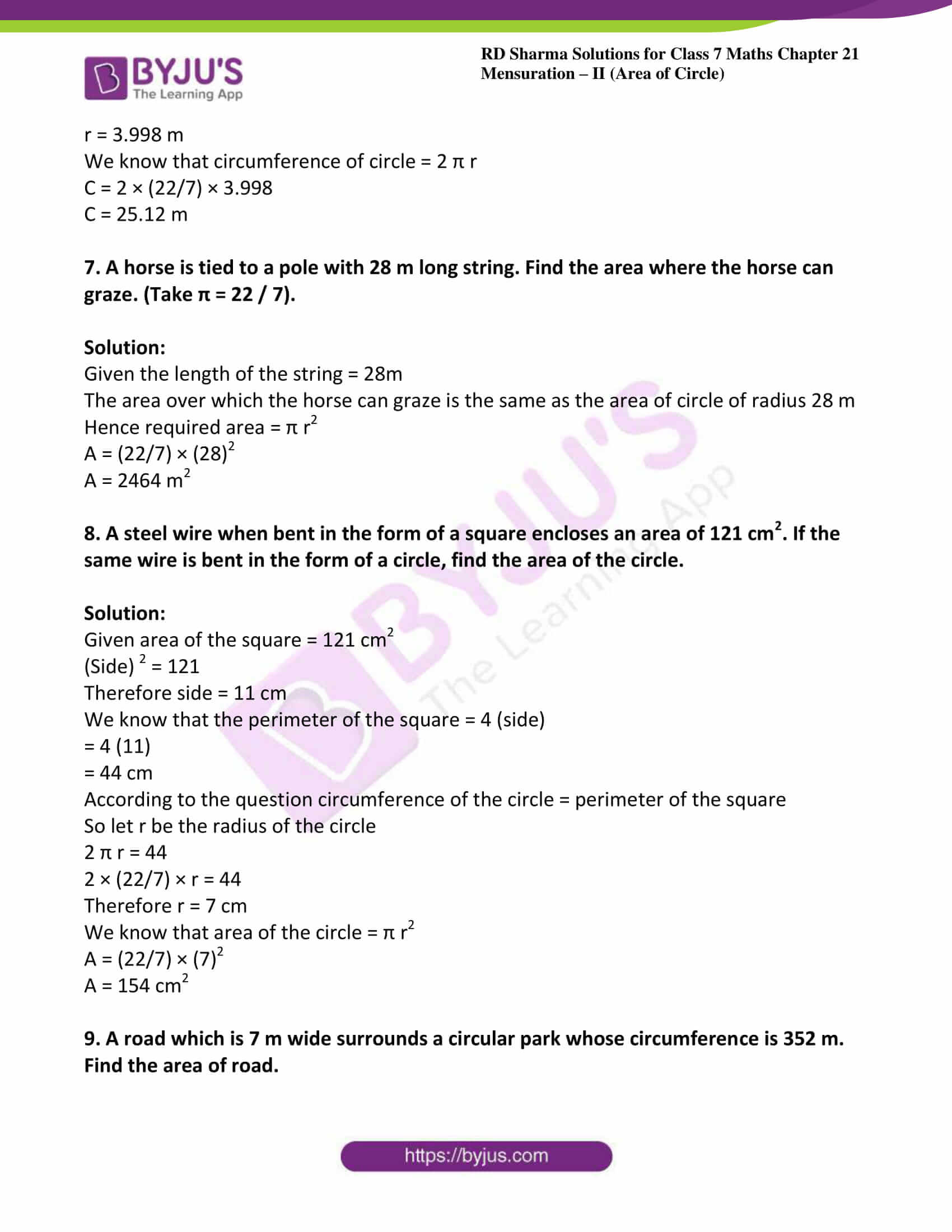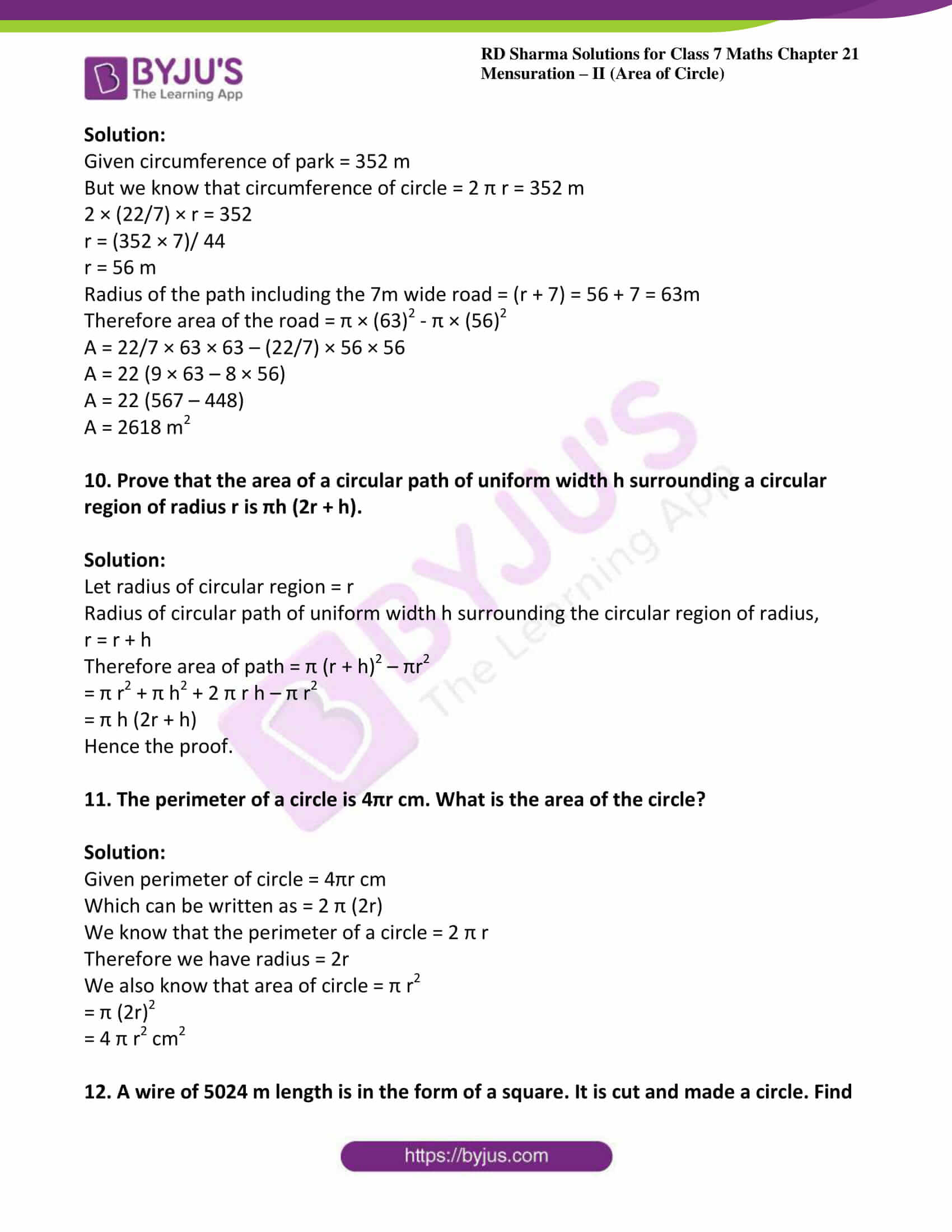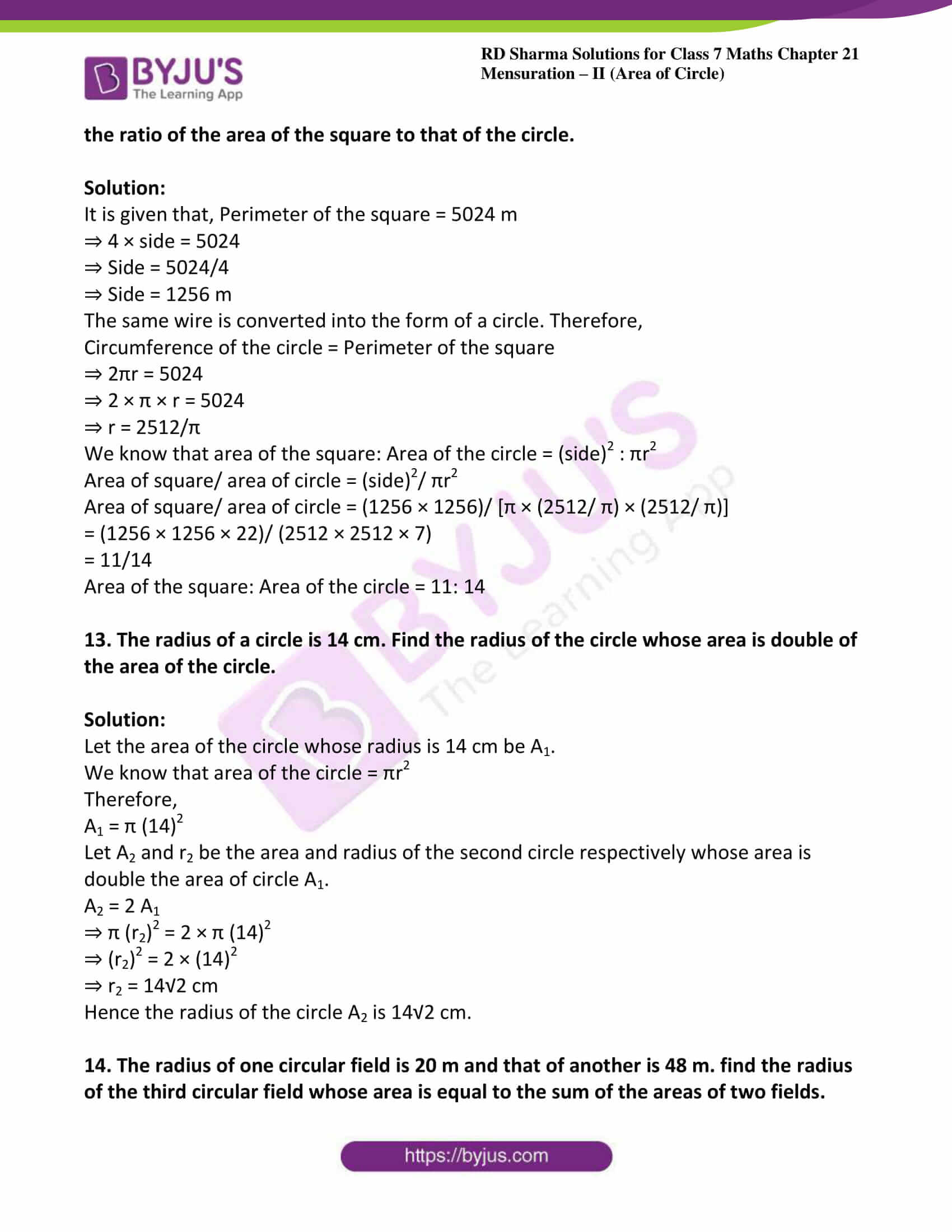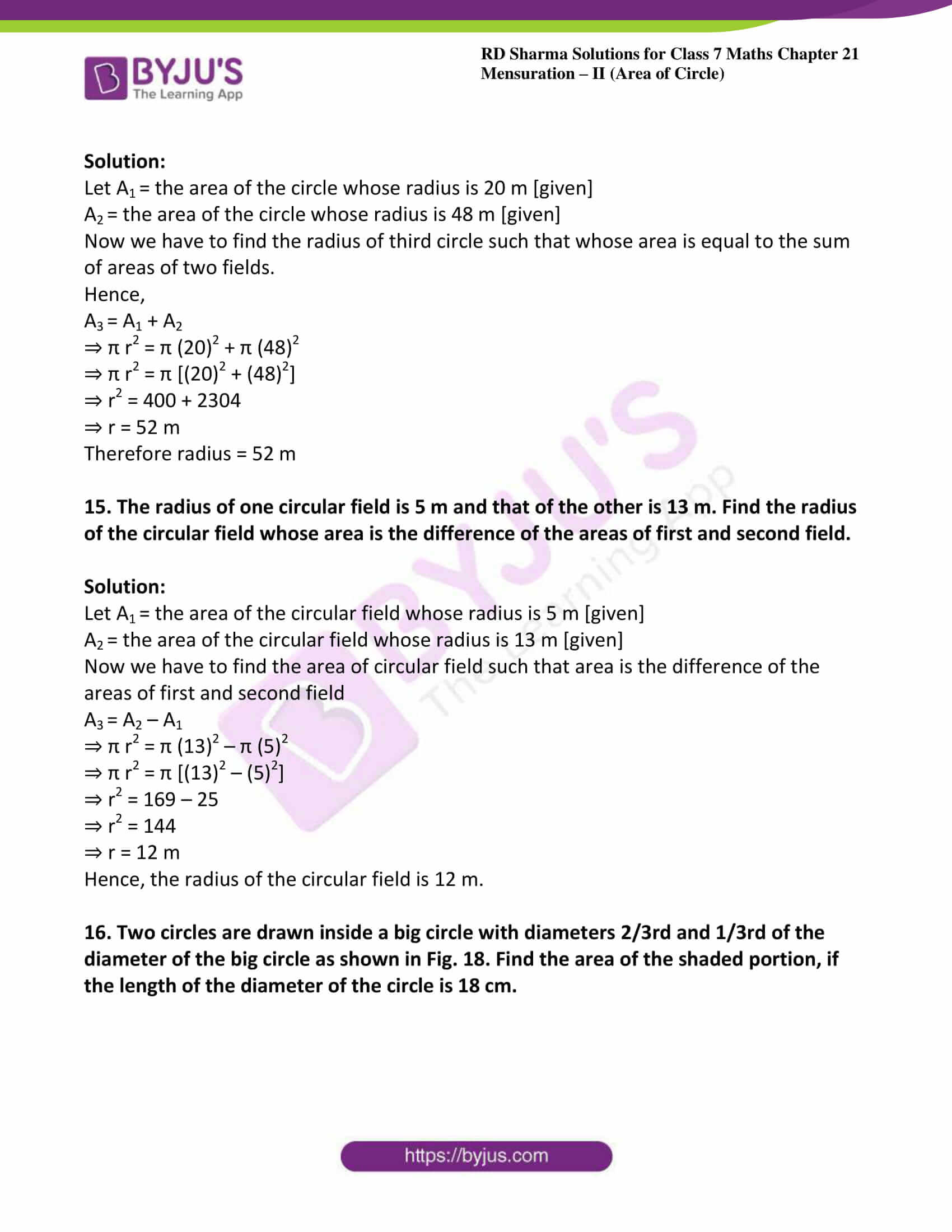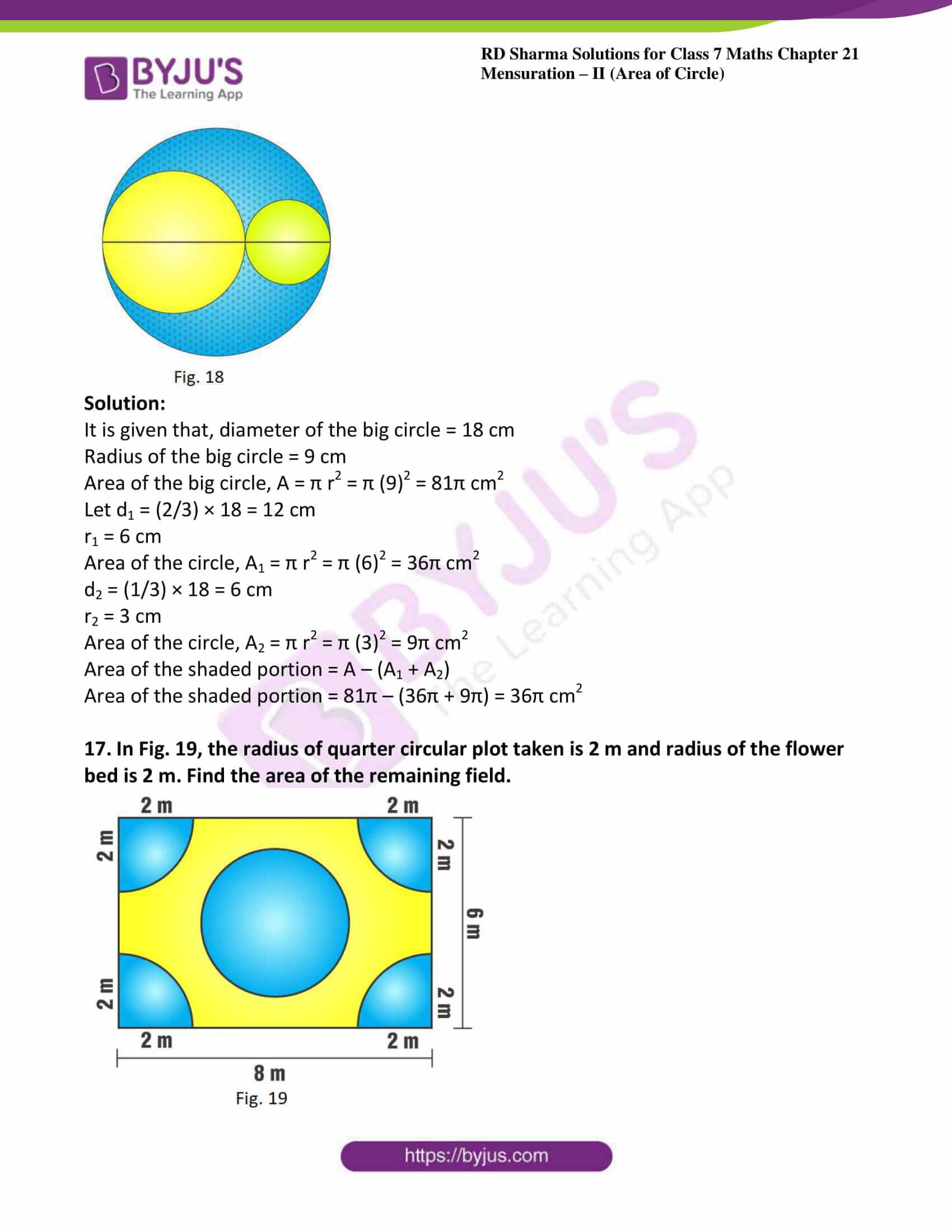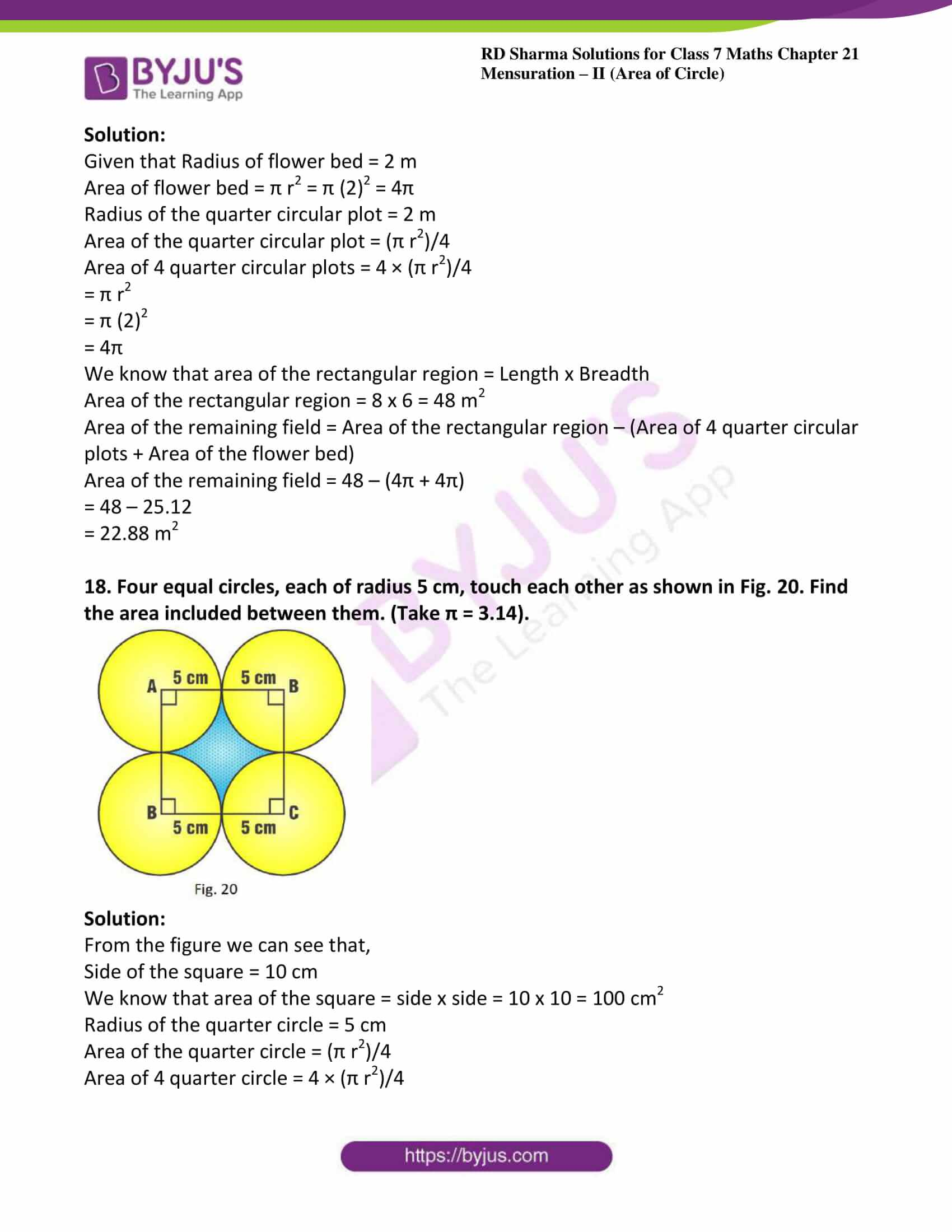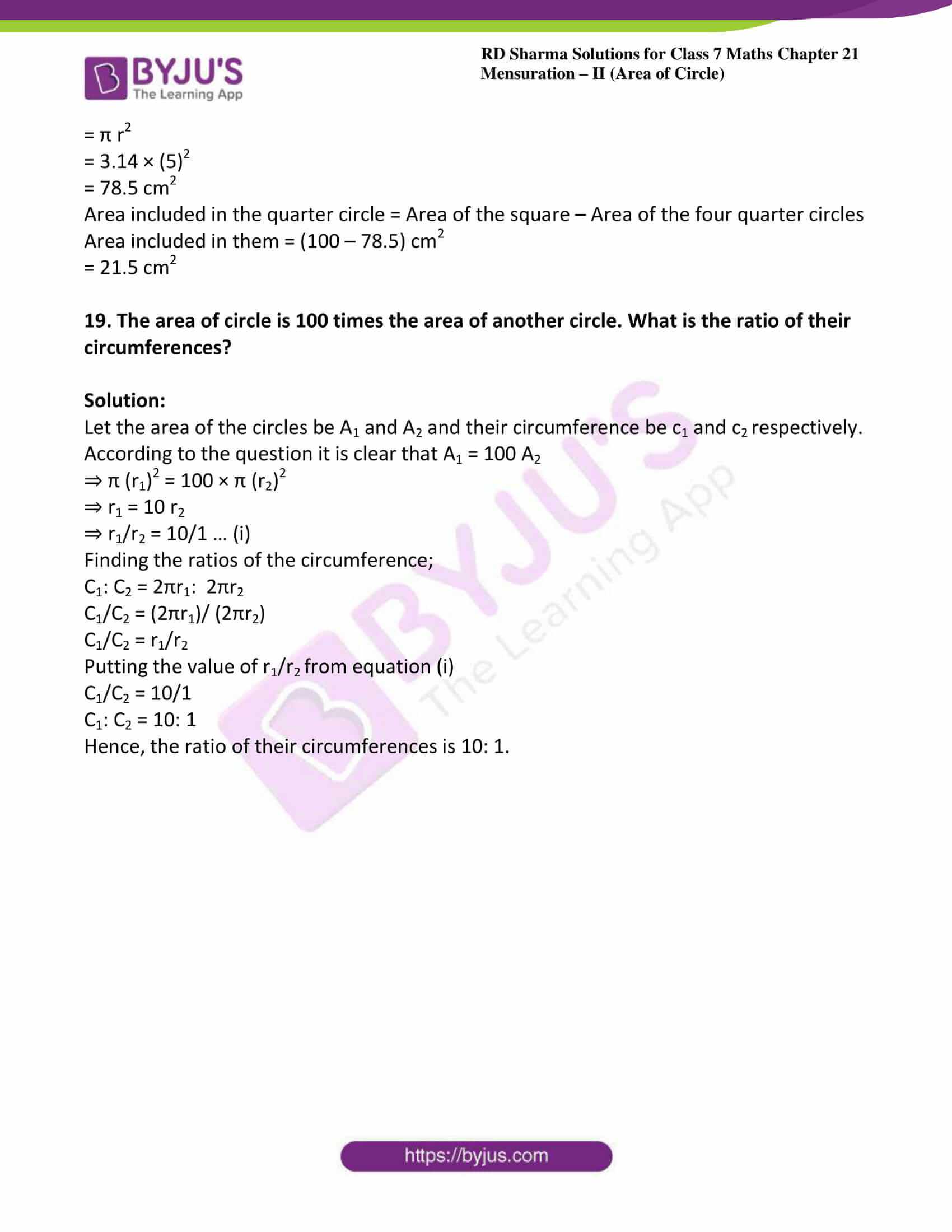### Access answers to Maths RD Sharma Solutions For Class 7 Chapter 21 – Mensuration – II (Area of circle)

Exercise 21.1 Page No: 21.7

1. Find the circumference of the circle whose radius is

(i) 14cm

(ii) 10m

(iii) 4km

Solution:

We know that the circumference of the circle = 2 π r

π value is 22/7

C = 2 × (22/7) × 14

C = 88cm

We know that the circumference of the circle = 2 π r

C = 2 × (22/7) × 10

C = 62.86 m

We know that the circumference of the circle = 2 π r

C = 2 × (22/7) × 4

C = 25.142 km

2. Find the circumference of a circle whose diameter is

(i) 7 cm

(ii) 4.2 cm

(iii) 11.2 km

Solution:

(i) Given diameter = 7cm

We know that radius = diameter/2

Therefore, r = d/2

r = 7/2

We know that the circumference of the circle = 2 π r

C = 2 × (22/7) × 7/2

C = 22 cm

(ii) Given diameter = 4.2 cm

We know that radius = diameter/2

Therefore, r = 4.2/2

r = 2.1

We know that the circumference of the circle = 2 π r

C = 2 × (22/7) × 2.1

C = 13.2 cm

(iii) Given diameter = 11.2 km

We know that radius = diameter/2

Therefore, r = 11.2/2

r = 5.6

We know that the circumference of the circle = 2 π r

C = 2 × (22/7) × 5.6

C = 35.2 km

3. Find the radius of a circle whose circumference is

(i) 52.8 cm

(ii) 42 cm

(iii) 6.6 km

Solution:

(i) Given circumference, C = 52.8 cm

We know that the circumference of the circle = 2 π r

Therefore radius, r = C/2 π

r = (52.8 × 7)/ (2 × 22)

r = 369.6/ 44

r = 8.4 cm

(ii) Given circumference, C = 42 cm

We know that the circumference of the circle = 2 π r

Therefore radius, r = C/2 π

r = (42 × 7)/ (2 × 22)

r = 294/ 44

r = 6.68 cm

(iii) Given circumference, C = 6.6 km

We know that the circumference of the circle = 2 π r

Therefore radius, r = C/2 π

r = (6.6 × 7)/ (2 × 22)

r = 46.2/ 44

r = 1.05 km

4. Find the diameter of a circle whose circumference is

(i) 12.56 cm

(ii) 88 m

(iii) 11.0 km

Solution:

(i) Given circumference, C = 12.56 cm

We know that the circumference of the circle = 2 π r

Therefore radius, r = C/2 π

r = (12.56 × 7)/ (2 × 22)

r = 87.92/ 44

r = 1.99 cm

But diameter = 2r

= 2 × 1.99 = 3.99 cm

(ii) Given circumference, C = 88 m

We know that the circumference of the circle = 2 π r

Therefore radius, r = C/2 π

r = (88 × 7)/ (2 × 22)

r = 616/ 44

r = 14 m

But diameter = 2r

= 2 × 14 = 28 m

(iii) Given circumference, C = 11.0 km

We know that the circumference of the circle = 2 π r

Therefore radius, r = C/2 π

r = (11 × 7)/ (2 × 22)

r = 77/ 44

r = 1.75 km

But diameter = 2r

= 2 × 1.75 = 3.5 km

5. The ratio of the radii of two circles is 3: 2. What is the ratio of their circumferences?

Solution:

Given that the ratio of the radii = 3: 2

So, let the radii of the two circles be 3r and 2r respectively.

And let C1 and C2 be the circumference of the two circles of radii 3r and 2r respectively.

C1 = 2 π × 3r = 6 π r … (i)

Now C2 = 2 × 2 π r = 4 π r … (ii)

Consider, C1/C2 = (6 π r)/ 4 π r = 6/4 = 3/2

C1: C2 = 3: 2

6. A wire in the form of a rectangle 18.7 cm long and 14.3 cm wide is reshaped and bent into the form of a circle. Find the radius of the circle so formed.

Solution:

Given length of rectangular wire = 18.7 cm

Breadth of rectangular wire = 14.3 cm

According to the question length of wire = perimeter of the rectangle

= 2 (l + b) = 2 × (18.7 + 14.3)

= 2 (33)

= 66 cm

Let the wire bent in the form of circle of radius r cm then we have

Circumference = 66cm

2 π r = 66

2 × (22/7) × r = 66

(44/7) r = 66

r = (66 × 7)/44

r = 462/44

= 10.5 cm

7. A piece of wire is bent in the shape of an equilateral triangle of each side 6.6 cm. It is re-bent to form a circular ring. What is the diameter of the ring?

Solution:

Given side of equilateral triangle = 6.6 cm

Length of the wire = the perimeter of equilateral triangle

Perimeter of equilateral triangle = 3 × side

= 3 × 6.6 = 19.8 cm

Therefore circumference = 19.8 cm

C = 2 π r

19.8 = 2 × (22/7) × r

19.8 = (44/7) r

r = (19.8 × 7)/44

r = 138.6/44

= 3.15 cm

Diameter = 2r

Therefore diameter of ring = 2 × 3.15 = 6.3 cm

8. The diameter of a wheel of a car is 63 cm. Find the distance travelled by the car during the period, the wheel makes 1000 revolutions.

Solution:

It may be noted that in one revolution, the cycle covers a distance equal to the circumference of the wheel.

Given the diameter of the wheel = 63 cm

We know that circumference of the wheel = π d

= 22/7 x 63

= 198 cm.

Thus, the cycle covers 198 cm in one revolution.

Therefore the distance covered by the cycle in 1000 revolutions = (198 x 1000)

= 198000 cm

= 1980 m.

9. The diameter of a wheel of a car is 98 cm. How many revolutions will it make to travel 6160 meters.

Solution:

In one revolution of the wheel, the car travels a distance equal to the circumference of the wheel.

Given diameter of the wheel of a car = 98 cm

Circumference of the wheel of the car = π d

= 22/7 x 98

= 308 cm

The distance travelled by the car in one revolution = 308 cm

Total distance travelled by the car = 6160 m = 616000 cm

Therefore number of revolution = total distance travelled by the car/ distance travelled by the car in one revolution

Number of revolution = 616000/308 = 2000

10. The moon is about 384400 km from the earth and its path around the earth is nearly circular. Find the circumference of the path described by the moon in lunar month.

Solution:

From the question it is given that,

The radius of the path described by the moon around the earth = 384400 km

The circumference of the path described by the moon,

C = 2 π r

C = 2 × (22/7) × 384400

C = 2416228.57 km

11. How long will John take to make a round of a circular field of radius 21 m cycling at the speed of 8 km/hr.?

Solution:

Given the radius of the circular field = 21 m

Circumference of the circular field = 2 π r

C = 2 × (22/7) × 21

C = 132 m

If John cycles at a speed of 8 km/hr then John covers 8000 m in 1 hour.

(In 1 hour John covers 8 km = 8000 m)

So, time required to cover 132 m = 132/8000 = 0.0165 hours

As, 1 hour = 3600 seconds

By converting 0.0165 hours into minutes we get

0.0615 hours = 0.0165 x 3600 = 59.4 seconds.

12. The hour and minute hands of a clock are 4 cm and 6 cm long respectively. Find the sum of the distances travelled by their tips in 2 days.

Solution:

Length of the hour hand is 4 cm, which describes the radius of the path inscribed by the hour hand.

Length of the minute hand is 6 cm, which describes the radius of the path inscribed by the minute hand.

The circumference of the path inscribed by the hour hand = 2 π r

C = 2 × (22/7) × 4

C = 176/7 cm

The hour hand makes 2 revolutions in one day.

Therefore distance covered by the hour hand in 2 days = (176/7) × 2 × 2

= 100.57 cm

The distance covered by the minute hand in 1 revolution = 2 π r

C = 2 × (22/7) × 6

C = 264/7

As we know, the minute hand makes 1 revolution in one hour.

In 1 day, it makes 24 revolutions.

In 2 days, it makes 2 × 24 revolutions.

The distance covered by the minute hand in 2 days = 2 × 24 × (264/7)

= 12672/7

= 1810.28 cm

The sum of the distances travelled by the hour and minute hands in 2 days = 1810.28 + 100.57

= 1910.85 cm

13. A rhombus has the same perimeter as the circumference of the circle. If the side of the rhombus is 2.2m, find the radius of the circle.

Solution:

Given the side of a rhombus = 2.2 m

We know that the perimeter of the rhombus = 4 x side

= 4 x 2.2 m

= 8.8 m.

According to the question it is clear that,

Perimeter of the rhombus = Circumference of the circle

8.8 = 2 π r

8.8 = 2 × (22/7) × r

r = (8.8 × 7)/ 44

r = 61.6/44

r = 1.4 m

Therefore radius of the circle = 1.4m

14. A wire is looped in the form of a circle of radius 28 cm. It is re-bent into a square form. Determine the length of the side of the square.

Solution:

Given the radius of the circle = 28 cm

Using the circumference of the circle formula, we have

Circumference = 2 π r

C = 2 × (22/7) × 28

C = 176 cm

Let x cm be the side of the square. Then,

The circumference of the circle = the perimeter of the square

Perimeter of square = 4x

176 = 4 × x

x = 176/4

x = 44

Therefore the side of the square = 44 cm

15. A bicycle wheel makes 5000 revolutions in moving 11 km. Find the diameter of the wheel.

Solution:

Given total distance covered by bicycle in 5000 revolutions = 11 km = 11000 m

Therefore distance covered in 1 revolution = 11000/5000 = 2.2 m = 11/5

Distance covered in 1 revolution = Circumference of the wheel

C = π d

11/5 = (22/7) d

d = (11 × 7)/ (5 × 22)

d = 77/110

d = 0.7 m

Therefore diameter of wheel = 0.7 m = 70 cm

16. A boy is cycling such that the wheels of the cycle are making 140 revolutions per minute. If the diameter of the wheel is 60 cm, calculate the speed per hour with which the boy is cycling.

Solution:

Given the diameter of the wheel = 60 cm

Distance covered by the wheel in 1 revolution = Circumference of the wheel

Distance covered by the wheel in 1 revolution = π d

= 22/7 × 60 cm

Distance covered by the wheel in 140 revolutions = 22/7 × 60 × 140

= 26400 cm

Thus, the wheel covers 26400 cm in 1 minute. Then,

Speed = 26400/100 × 60 m/hr

= 264 × 60 m/hr

= 264 × 60/1000 km/hr

= 15.84 km/hr

The speed with which the boy is cycling is 15.84 km/hr.

17. The diameter of the driving wheel of a bus is 140 cm. How many revolutions per minute must the wheel make in order to keep a speed of 66 km per hour?

Solution:

Given diameter of the wheel = 140 cm

Desired speed of the bus = 66 km/hr

Distance covered by the wheel in 1 revolution = Circumference of the wheel

Circumference of the wheel = π d

C = 22/7 × 140

C = 440 cm

Now, the desired speed of the bus = 66 km/hr = (66 × 1000 × 100)/60 = 110000 cm/min

Number of revolution per minute = 110000/440

= 250

Therefore, the bus must make 250 revolutions per minute to keep the speed at 66 km/hr.

18. A water sprinkler in a lawn sprays water as far as 7 m in all directions. Find the length of the outer edge of wet grass.

Solution:

From the question it is clear that, a water sprinkler in a lawn sprays water as far as 7 m in all directions. So wet area shows a circular region of radius 7 m.

The length of the outer edge of the wet grass = Circumference of circle

Circumference of the circle = 2 π r

C = 2 × 22/7 × 7

C = 44 m

19. A well of diameter 150 cm has a stone parapet around it. If the length of the outer edge of the parapet is 660 cm. then find the width of the parapet.

Solution:

Given diameter of the well = 150 cm

Length of the outer edge of the parapet = 660 cm

Now we have to find width of the parapet

Now, radius of well = half of diameter = 150/2 = 75 cm

Consider the width of the stone parapet be x cm. then, according to the question outer edge of the parapet forms a circular region of radius (x + 75) cm

So, 660 = 2 × 22/7 × (x + 75)

(660 × 7)/ 2 × 22 = x + 75

4620/44 = x + 75

105 = x + 75

x = 105 – 75

x = 30

The width of the parapet = x = 30cm

20. An ox in a kolhu (an oil processing apparatus) is tethered to a rope 3 m long. How much distance does it cover in 14 rounds?

Solution:

Radius of the circular path traced by the ox = 3 m and

Distance covered by an ox in 1 round = Circumference of the circular path

Circumference = 2 π r

C = 2 × (22/7) × 3 m

Distance covered in 14 rounds = 2 × (22/7) × 3 × 14

C = 264 m

Exercise 21.2 Page No: 21.15

1. Find the area of a circle whose radius is

(i) 7 cm

(ii) 2.1 m

(iii) 7 km

Solution:

(i) Given radius = 7 cm

We know that area of circle = π r2

A = (22/7) × 72

A = 22 × 7

A = 154 cm2

(ii) Given radius = 2.1 m

We know that area of circle = π r2

A = (22/7) × (2.1)2

A = 22/7 × 4.41

A = 13.86 m2

(iii) Given radius = 7 km

We know that area of circle = π r2

A = (22/7) × 72

A = 22 × 7

A = 154 km2

2. Find the area of a circle whose diameter is

(i) 8.4 cm

(ii) 5.6 m

(iii) 7 km

Solution:

(i) Given diameter = 8.4 cm

Therefore radius, r = d/2 = 8.4/2 = 4.2

We know that area of circle = π r2

A = (22/7) × (4.2)2

A = 55.44 cm2

(ii) Given diameter = 5.6 cm

Therefore radius, r = d/2 = 5.6/2 = 2.8

We know that area of circle = π r2

A = (22/7) × (2.8)2

A = 24.64 cm2

(iii) Given diameter = 7 km

Therefore radius, r = d/2 = 7/2 = 3.5

We know that area of circle = π r2

A = (22/7) × (3.5)2

A = 38.5 km2

3. The area of a circle is 154 cm2. Find the radius of the circle.

Solution:

Given area of the circle = 154 cm2

A = π r2

154 = 22/7 r2

r2 = (154 × 7)/22

r2 = 49

r = 7 cm

4. Find the radius of a circle, if its area is

(i) 4 π cm2

(ii) 55.44 m2

(iii) 1.54 km2

Solution:

(i) We know that area of circle = π r2

Given area = 4 π cm2

A = π r2

4 π = π r2

r2 = 4

Therefore r = 2 cm

(ii) We know that area of circle = π r2

Given area = 55.44 cm2

A = π r2

55.44 = π r2

r2 = (55.44 × 7)/22

r2 = 17.64 m

r = 4.2 m

(iii) We know that area of circle = π r2

Given area = 1.54 km2

A = π r2

1.54 = π r2

r2 = (1.54 × 7)/22

r2 = 0.49 km

r = 0.7 km

r = 700m

5. The circumference of a circle is 3.14 m, find its area.

Solution:

Given circumference = 3.14m

We know that circumference of circle = 2 π r

3.14 = 2 × 3.14 × r

r = 3.14/ (2 × 3.14)

r = 0.5

We know that area of circle = π r2

A = (22/7) × (0.5)2

A = 0.785 m2

6. If the area of a circle is 50.24 m2, find its circumference.

Solution:

Given area of a circle is 50.24 m2

We know that area of circle = π r2

50.24 = (22/7) × r2

r2 = (50.24 × 7)/22

r2 = 15.985

r = 3.998 m

We know that circumference of circle = 2 π r

C = 2 × (22/7) × 3.998

C = 25.12 m

7. A horse is tied to a pole with 28 m long string. Find the area where the horse can graze. (Take π = 22 / 7).

Solution:

Given the length of the string = 28m

The area over which the horse can graze is the same as the area of circle of radius 28 m

Hence required area = π r2

A = (22/7) × (28)2

A = 2464 m2

8. A steel wire when bent in the form of a square encloses an area of 121 cm2. If the same wire is bent in the form of a circle, find the area of the circle.

Solution:

Given area of the square = 121 cm2

(Side) 2 = 121

Therefore side = 11 cm

We know that the perimeter of the square = 4 (side)

= 4 (11)

= 44 cm

According to the question circumference of the circle = perimeter of the square

So let r be the radius of the circle

2 π r = 44

2 × (22/7) × r = 44

Therefore r = 7 cm

We know that area of the circle = π r2

A = (22/7) × (7)2

A = 154 cm2

9. A road which is 7 m wide surrounds a circular park whose circumference is 352 m. Find the area of road.

Solution:

Given circumference of park = 352 m

But we know that circumference of circle = 2 π r = 352 m

2 × (22/7) × r = 352

r = (352 × 7)/ 44

r = 56 m

Radius of the path including the 7m wide road = (r + 7) = 56 + 7 = 63m

Therefore area of the road = π × (63)2 – π × (56)2

A = 22/7 × 63 × 63 – (22/7) × 56 × 56

A = 22 (9 × 63 – 8 × 56)

A = 22 (567 – 448)

A = 2618 m2

10. Prove that the area of a circular path of uniform width h surrounding a circular region of radius r is πh (2r + h).

Solution:

Let radius of circular region = r

Radius of circular path of uniform width h surrounding the circular region of radius,

r = r + h

Therefore area of path = π (r + h)2 – πr2

= π r2 + π h2 + 2 π r h – π r2

= π h (2r + h)

Hence the proof.

11. The perimeter of a circle is 4πr cm. What is the area of the circle?

Solution:

Given perimeter of circle = 4πr cm

Which can be written as = 2 π (2r)

We know that the perimeter of a circle = 2 π r

Therefore we have radius = 2r

We also know that area of circle = π r2

= π (2r)2

= 4 π r2 cm2

12. A wire of 5024 m length is in the form of a square. It is cut and made a circle. Find the ratio of the area of the square to that of the circle.

Solution:

It is given that, Perimeter of the square = 5024 m

⇒ 4 × side = 5024

⇒ Side = 5024/4

⇒ Side = 1256 m

The same wire is converted into the form of a circle. Therefore,

Circumference of the circle = Perimeter of the square

⇒ 2πr = 5024

⇒ 2 × π × r = 5024

⇒ r = 2512/π

We know that area of the square: Area of the circle = (side)2 : πr2

Area of square/ area of circle = (side)2/ πr2

Area of square/ area of circle = (1256 × 1256)/ [π × (2512/ π) × (2512/ π)]

= (1256 × 1256 × 22)/ (2512 × 2512 × 7)

= 11/14

Area of the square: Area of the circle = 11: 14

13. The radius of a circle is 14 cm. Find the radius of the circle whose area is double of the area of the circle.

Solution:

Let the area of the circle whose radius is 14 cm be A1.

We know that area of the circle = πr2

Therefore,

A1 = π (14)2

Let A2 and r2 be the area and radius of the second circle respectively whose area is double the area of circle A1.

A2 = 2 A1

⇒ π (r2)2 = 2 × π (14)2

⇒ (r2)2 = 2 × (14)2

⇒ r2 = 14√2 cm

Hence the radius of the circle A2 is 14√2 cm.

14. The radius of one circular field is 20 m and that of another is 48 m. find the radius of the third circular field whose area is equal to the sum of the areas of two fields.

Solution:

Let A= the area of the circle whose radius is 20 m [given]

A= the area of the circle whose radius is 48 m [given]

Now we have to find the radius of third circle such that whose area is equal to the sum of areas of two fields.

Hence,

A= A1 + A2

⇒ π r2 = π (20)2 + π (48)2

⇒ π r2 = π [(20)2 + (48)2]

⇒ r2 = 400 + 2304

⇒ r = 52 m

15. The radius of one circular field is 5 m and that of the other is 13 m. Find the radius of the circular field whose area is the difference of the areas of first and second field.

Solution:

Let A= the area of the circular field whose radius is 5 m [given]

A= the area of the circular field whose radius is 13 m [given]

Now we have to find the area of circular field such that area is the difference of the areas of first and second field

A= A2 – A1

⇒ π r2 = π (13)2 – π (5)2

⇒ π r2 = π [(13)2 – (5)2]

⇒ r2 = 169 – 25

⇒ r2 = 144

⇒ r = 12 m

Hence, the radius of the circular field is 12 m.

16. Two circles are drawn inside a big circle with diameters 2/3rd and 1/3rd of the diameter of the big circle as shown in Fig. 18. Find the area of the shaded portion, if the length of the diameter of the circle is 18 cm.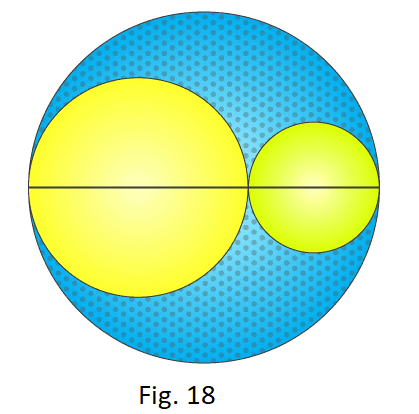Solution:

It is given that, diameter of the big circle = 18 cm

Radius of the big circle = 9 cm

Area of the big circle, A = π r2 = π (9)2 = 81π cm2

Let d1 = (2/3) × 18 = 12 cm

r1 = 6 cm

Area of the circle, A1 = π r2 = π (6)2 = 36π cm2

d2 = (1/3) × 18 = 6 cm

r2 = 3 cm

Area of the circle, A2 = π r2 = π (3)2 = 9π cm2

Area of the shaded portion = A – (A1 + A2)

Area of the shaded portion = 81π – (36π + 9π) = 36π cm2

17. In Fig. 19, the radius of quarter circular plot taken is 2 m and radius of the flower bed is 2 m. Find the area of the remaining field.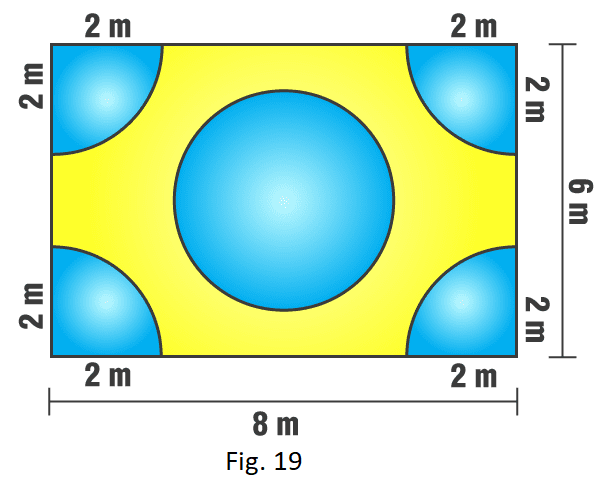Solution:

Given that Radius of flower bed = 2 m

Area of flower bed = π r2 = π (2)2 = 4π

Radius of the quarter circular plot = 2 m

Area of the quarter circular plot = (π r2)/4

Area of 4 quarter circular plots = 4 × (π r2)/4

= π r

= π (2)2

= 4π

We know that area of the rectangular region = Length x Breadth

Area of the rectangular region = 8 x 6 = 48 m2

Area of the remaining field = Area of the rectangular region – (Area of 4 quarter circular plots + Area of the flower bed)

Area of the remaining field = 48 – (4π + 4π)

= 48 – 25.12

= 22.88 m2

18. Four equal circles, each of radius 5 cm, touch each other as shown in Fig. 20. Find the area included between them. (Take π = 3.14).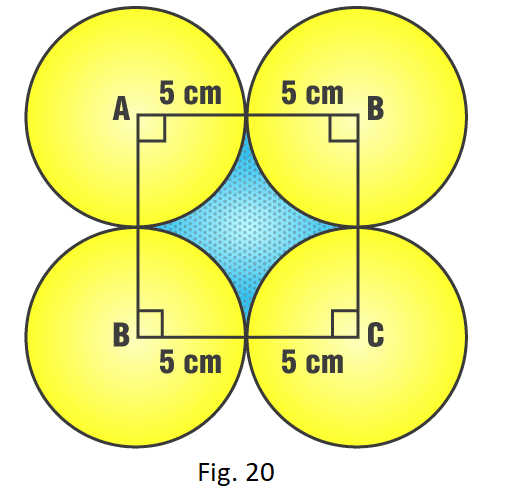Solution:

From the figure we can see that,

Side of the square = 10 cm

We know that area of the square = side x side = 10 x 10 = 100 cm2

Radius of the quarter circle = 5 cm

Area of the quarter circle = (π r2)/4

Area of 4 quarter circle = 4 × (π r2)/4

= π r

= 3.14 × (5)2

= 78.5 cm2

Area included in the quarter circle = Area of the square – Area of the four quarter circles

Area included in them = (100 – 78.5) cm

= 21.5 cm2

19. The area of circle is 100 times the area of another circle. What is the ratio of their circumferences?

Solution:

Let the area of the circles be A1 and A2 and their circumference be c1 and crespectively.

According to the question it is clear that A1 = 100 A2

⇒ π (r1)2 = 100 × π (r2)2

⇒ r1 = 10 r2

⇒ r1/r2 = 10/1 … (i)

Finding the ratios of the circumference;

C1: C2 = 2πr1:  2πr2

C1/C2 = (2πr1)/ (2πr2)

C1/C2 = r1/r2

Putting the value of r1/rfrom equation (i)

C1/C2 = 10/1

C1: C2 = 10: 1

Hence, the ratio of their circumferences is 10: 1.